• matlab读取csv文件数据并绘图

万次阅读 2018-05-11 21:18:00
Matlab提供了一些绘图选项，用于确定所绘曲线的线型、颜色和数据点标记符号。这些选项如表所示： 线型 颜色 标记符号 - 实线 b蓝色 ...

circle.m（画二维圆的函数）

%该函数是画二维圆圈，输入圆心坐标和半径
%rectangle()函数参数‘linewidth’修饰曲线的宽度
%'edgecolor'，'r'，edgecolor表示边框颜色，r表示颜色参数
%'facecolor','b'，facecolor表示内部填充颜色，b表示颜色参数
function [] = circle( x,y,r )
rectangle('Position',[x-r,y-r,2*r,2*r],'Curvature',[1,1],'linewidth',1);
axis equal;
end

draw_arrow.m（画两坐标点之间箭头的函数）

%该函数是画从一坐标点到另一坐标点的指向箭头

function draw_arrow(start_point, end_point)
% 从start_point到end_point画一箭头

K = 0.05;  %箭头比例系数

theta = pi / 8;  %箭头角度
A1 = [cos(theta), -sin(theta);
sin(theta), cos(theta)];  %旋转矩阵
theta = -theta;
A2 = [cos(theta), -sin(theta);
sin(theta), cos(theta)];  %旋转矩阵

arrow = start_point' - end_point';

arrow_1 = A1 * arrow;
arrow_2 = A2 * arrow;
arrow_1 = K * arrow_1 + end_point';
arrow_2 = K * arrow_2 + end_point';

hold on;
grid on;
axis equal;
plot([start_point(1), end_point(1)], [start_point(2), end_point(2)], 'k');
plot([arrow_1(1), end_point(1)], [arrow_1(2), end_point(2)], 'k');
plot([arrow_2(1), end_point(1)], [arrow_2(2), end_point(2)], 'k');
hold off;

clear;
close all;
clc;
%importdata 函数允许加载各种数据的不同格式的文件
data=importdata('data.csv'); %读取csv数据文件
%disp(data); %disp函数：显示文本或数组
for i=1:75
if i<=7
x=data(i,1);
y=data(i,2);
plot(x,y,'rs'),axis([0 400 0 800]);
hold on;
else
x=data(i,1);
y=data(i,2);
plot(x,y,'g.'),axis([0 400 0 800]);
hold on;
end
end
xlabel('x/km'),ylabel('y/km'); %添加标签
hold on;
%grid on;%添加网格
circle(data(8,1),data(8,2),70);   %调用画圆圈的函数
circle(data(18,1),data(18,2),70);
circle(data(27,1),data(27,2),70);
circle(data(32,1),data(32,2),70);
circle(data(42,1),data(42,2),70);
circle(data(49,1),data(49,2),70);
circle(data(55,1),data(55,2),70);
circle(data(61,1),data(61,2),70);
circle(data(66,1),data(66,2),70);
circle(data(71,1),data(71,2),70);
draw_arrow([data(55,1), data(55,2)],[data(66,1), data(66,2)]); %调用画箭头的函数
draw_arrow([data(3,1), data(3,2)],[data(66,1), data(66,2)]);
axis([-100 400 0 800]);
hold on;

结果为：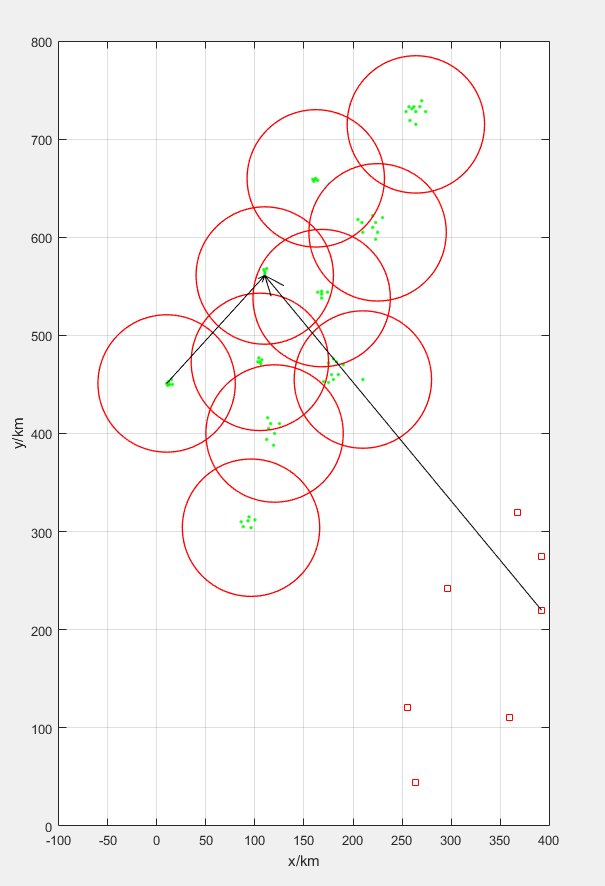data.csv数据如下(位置的坐标)：

368,319
264,44
392,220
360,110
392,275
296,242
256,121
264,715
258,719
274,728
264,728
254,728
257,733
260,731
262,733
268,733
270,739
225,605
223,598
210,605
220,610
223,615
209,615
230,620
220,622
205,618
168,538
168,542
164,544
168,545
174,544
210,455
180,455
175,452
170,453
185,460
178,460
190,470
183,473
175,472
180,476
120,400
119,388
112,394
125,410
114,405
116,410
113,416
96,304
88,305
100,312
93,311
86,310
94,315
10,451
11,449
13,450
16,450
12,453
15,455
162,660
161,659
159,659
160,657
164,658
110,561
110,563
110,565
109,567
112,568
105,473
106,471
103,473
107,475
104,477

附加：

plot函数

Matlab提供了一些绘图选项，用于确定所绘曲线的线型、颜色和数据点标记符号。这些选项如表所示：

 线型 颜色 标记符号 - 实线 b蓝色 .   点 s 方块 : 虚线 g绿色 o 圆圈 d 菱形 -. 点划线 r红色 × 叉号 ∨朝下三角符号 -- 双划线 c青色 + 加号 ∧朝上三角符号 m品红 * 星号 <朝左三角符号 y黄色 >朝右三角符号 k黑色 p 五角星 w白色 h 六角星

参考：

1、matlab详细绘图

2、matlab教程

展开全文matlab 文件处理
• # 导入csv文件中的第二行第三列的所有数据 N = csvread('kongA-Aref.csv', 1, 2); baseline_1 = mean(M(1:16)); # 取列表中数据的前十六个进行均值处理把它设置为基础值 baseline_2 = mean(M(1:16)); A = length...
clc;clear all
close all
M = csvread('shui_A-Aref.csv', 1, 2);  # 导入csv文件中的第二行第三列的所有数据
baseline_1 = mean(M(1:16));   # 取列表中数据的前十六个进行均值处理并把它设置为基础值
baseline_2 = mean(M(1:16));
A = length(M);  # 计算csv文件中第三列的数据的个数
B = length(N);
j = 0;
k = 0;
a = [];  # 建立一个空的矩阵，保存下列所产生的坐标
c = [];
threshold = -1050;  #设置一个阈值，用于对csv文件中数据的处理
% 将A数组的数据一个个输入
for i = 17:A    # 对csv中数据从第十七个到最后进行遍历，一个个输入
if M(i) < baseline_1  # 负值维护机制
baseline_1 = M(i);
j = 0;
elseif M(i)>baseline_1 && M(i) < threshold  # 缓慢维护机制
baseline_1 = baseline_1*0.75+0.25*M(i);
j = 0;
elseif  M(i)>=threshold
j = j+1 ;
if j >= 8
a = [a;i,M(i)]; # 如果连续的8个值都大于阈值，则取出第八个及以后的值的坐标，存在矩阵a[]中
fprintf('chufa\n')
end
end
end
% 将B数组的数据一个个输入
for  h = 17:B
if N(h) < baseline_2   # 负值维护
baseline_2 = N(h);
k=0;
elseif N(h)>baseline_2 && N(h)<threshold   # 缓慢维护
baseline_2 = baseline_2*0.75+0.25*N(h);
k = 0;
elseif  N(h)>=threshold  # 如果连续的8个值都大于阈值，则取出第八个及以后的值的坐标，存在矩阵c[]中
k = k+1 ;
if k >= 8
c = [c;h,N(h)];
fprintf("chufa\n")
end
end
end
# 保存坐标的目的是想在由N中数据所绘制的图上进行标点，记为触发点
plot(N)
hold on
scatter(c(:,1),c(:,2),'*')
hold off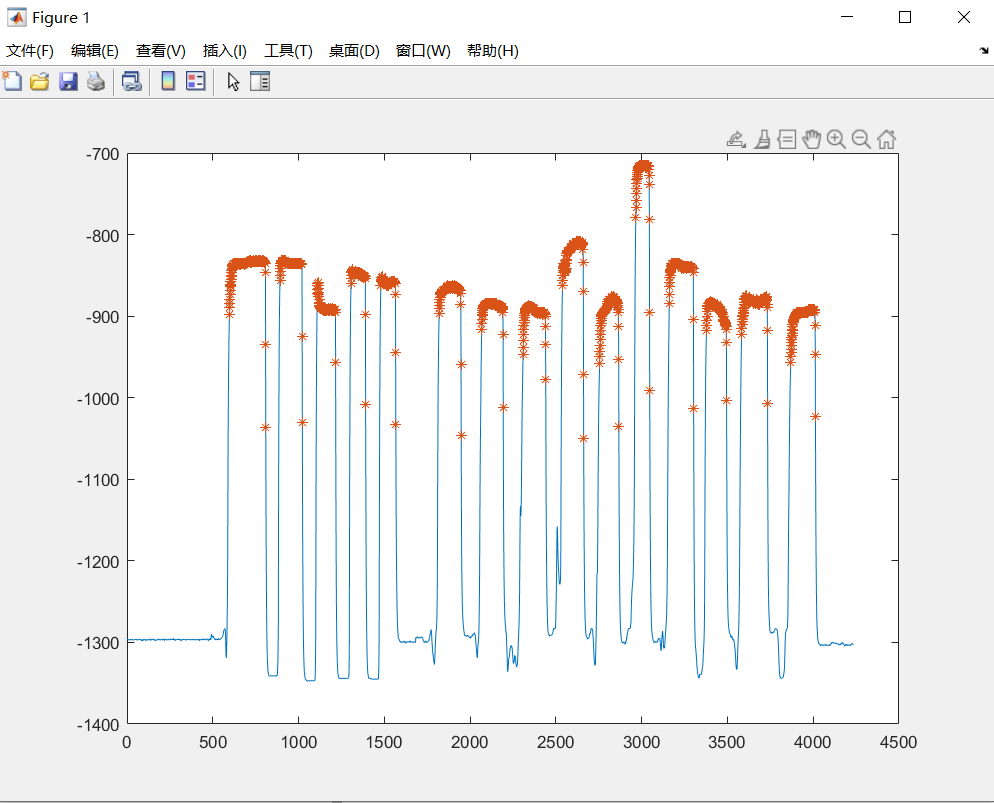展开全文matlab csv 矩阵 算法
• MATLAB | 读取.csv文件并绘图

千次阅读 2020-02-25 08:54:36
对本地的csv文件进行读取，格式转换，并绘图

一共分为三步：

1. 读取文件
2. 数据转换
3. 绘图

第一步：读取文件使用如下命令：

data=fopen('filename.csv');
a=textscan(data,'%s');
fclose(data);

这一步进行之后，csv中的数据被读入到一个元组中，可以去工作区查看里边的内容

第二部：数据转换，先将一个元组中的所有子元祖拿出来，才好用角标读取数据：

b=a{1};
fprintf('b的大小为：%d x %d \n',size(b,1),size(b,2))

然后对数据进行转换，因为是字符串，需要转换为数字格式

可以直接转为数字的字符串，使用如下命令：

c=str2double(b{i});

需要拆分的，使用split或者regexp函数

d=split(c,',');%以逗号为间隔拆分读取

f=regexp(e,':','split');以冒号为间隔拆分读取

第三步，在数据格式转换完成之后，就只剩下的绘图了

再次感谢学校，让我可以免费使用正版MATLAB

展开全文matlab
• 最近作实验须要使用matlab导入并处理csv文件数据，在此以前对matlab的使用不熟悉，因此趁着撰写博客的机会总结巩固一下。一、脚本程序清除命令：clc：清空命令行窗口的内容，对编辑器及工做区的内容无影响。close...

最近作实验须要使用matlab导入并处理csv文件数据，在此以前对matlab的使用并不熟悉，因此趁着撰写博客的机会总结巩固一下。

一、脚本程序清除命令：

clc：清空命令行窗口的内容，对编辑器及工做区的内容无影响。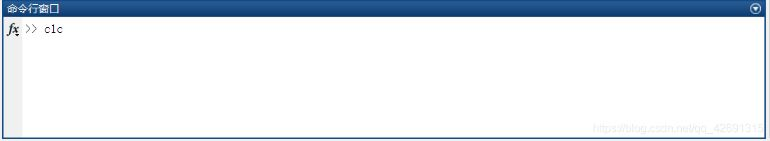close与close all：二者功能一致，前者是关闭当前Figure窗口；后者是关闭全部Figure窗口

clear与clear all：二者功能相近，前者是清除工做区的全部变量；后者是清除全部的变量、函数及运行文件等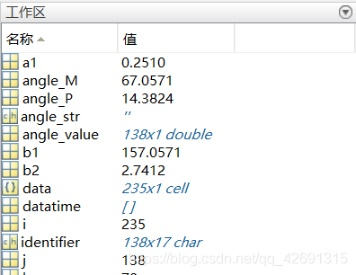通常在撰写脚本程序前，使用clc,clear all,close all命令，便可使程序运行前彻底复位。web

二、文件的导入处理：

importdata函数能够从txt、csv、excle及图片文件中导入数据，其经常使用的使用形式以下：正则表达式

data = importdata(filename)；

data = importdata(filename, delimiter)；

data = importdata(filename, delimiter, headerlinesIn )；express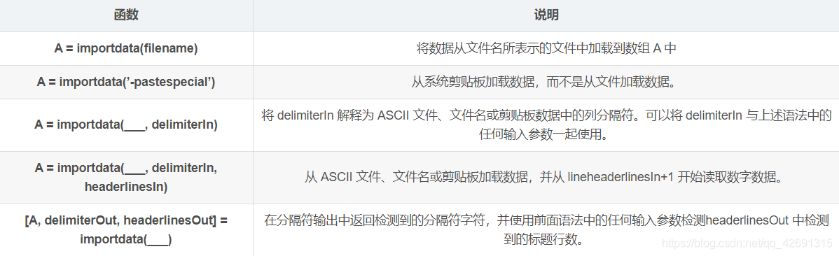三、文件数据查找匹配：

matlab提供了三个正则表达式函数：数组

regexp函数：用于对字符串进行查找，大小写敏感；

regexpi函数：用于对字符串进行查找，大小写不敏感；

regexprep函数：用于对字符串进行查找并替换编辑器

经常使用的函数形式以下：svg

regexp(str,expression,‘match’)； 从str字符串中匹配并返回expression表达形式的字符串，区分大小写。

regexp(str,expression,‘split’); 将str字符串按expression表达形式进行分割

regexpi(str,expression,‘match’)； 从str字符串中匹配并返回expression表达形式的字符串，不区分大小写。

regexprep(str,expression1,expression2); 将str字符串中的expression1表达式替换为expression2。函数

举例：

tmp=regexp(str, ‘,’ ,‘split’); %正则表达式，将str字符串按’，'进行分割，结果存在tmp中

regexp(str, ‘abc’, ‘match’)); %从str中开始匹配符合abc的表达式

regexprep(str, ‘abc’, ‘a’)); %将str中的’abc’字符替换为a命令行

注意：

. 表示匹配任何单字符(除换行符 \n 以外)，*表示贪婪模式。好比a.*b，它将会匹配字符串中以a开始，以b结束的最长的字符串。若是用它来搜索字符串“aabab”的话，它会匹配整个字符串aabab。这被称为贪婪匹配。code

四、图形绘制：

matlab中有丰富且强大的图形绘制函数，其中应用最普遍的为plot函数，plot函数的经常使用形式为plot(X)、plot(X,Y)、plot(X,Y，…)，其中XY为长度相同的向量，分别存储Figure图中的X轴与Y轴坐标。plot函数中能够添加各类绘图属性，好比：regexp

LineWidth——指定线宽

MarkerEdgeColor——指定标识符的边缘颜色

MarkerFaceColor——指定标识符填充颜色

MarkerSize——指定标识符的大小

注意上面四个属性是针对当前坐标系中全部曲线的。而针对绘图中的线型属性以下：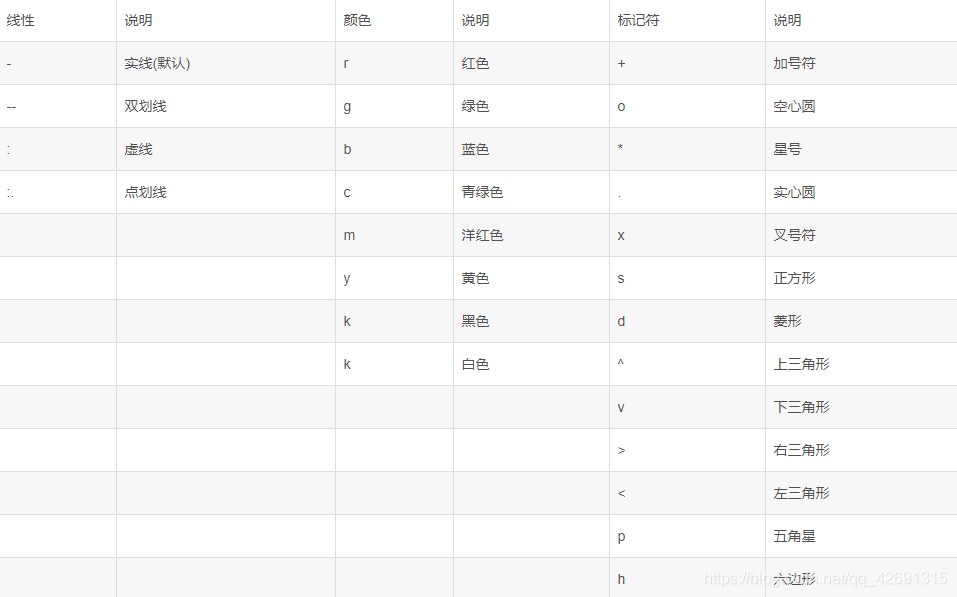其余的图形设置好比：

title(’Figure名称’)； 为图形加入标题

text(x，y，’图形说明’)； 在指定x、y坐标加入说明

xlabel(’x轴说明’)；

ylabel(’y轴说明’)；

axis on/off ； 显示/取消坐标轴

axis equal； xy轴上的各个刻度线的增量相同

grid on/off 开启/关闭坐标轴网格线

示例：

point_X=[x1,X_aim,x2]; %point_X=[0, 62.71, 100]

point_Y=[y1,Y_aim,y2];%point_Y=[0, 16.80, 0]

plot(point_X, point_Y, ':^r', 'markersize',8);%点线，上三角标记，红色，标记大小8

title('这是一张figure图');%标题

xlabel('x轴坐标');%轴加备注

ylabel('y轴坐标');

text(X_aim+2,Y_aim+1,'1');%指定坐标加备注

text(x1+2,y1,'2');

text(x2-4,y2,'3');

axis equal%xy轴上的各个刻度线的增量相同

grid on%坐标轴加网格线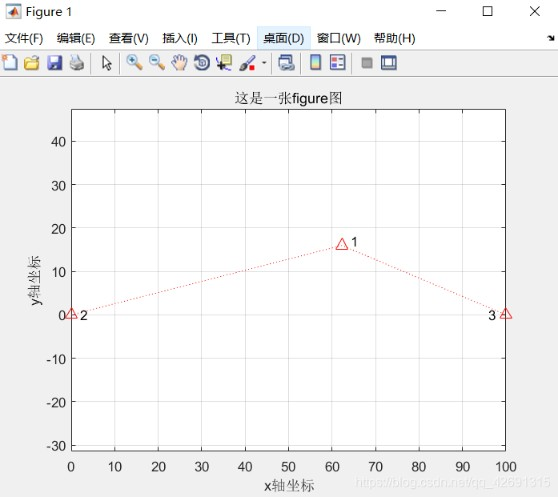展开全文• 最近做实验需要使用matlab导入并处理csv文件数据，而在此之前对matlab的使用不熟悉，所以在撰写博客的同时也借此机会总结一下。 1、脚本程序清除命令： clc：清空命令行窗口的内容，对编辑器及工作区的内容无影响...matlab 字符串 正则表达式
• 1、将.csv文件直接拖到MatLab的控制台区域，MatLab会直接打开.csv文件，就像EXCEL打开.csv一样MatLab会对每一列数据赋给一个列名称，默认值为：2、双击列标题，将列名称修改为数据的标题，3、在列标题上点击鼠标右键...MatLabmatlab 矩阵
• MATLAB2017b使用CSV文件的列数据画图 前天升级了MATLAB到2017b，发现在笔记一中刚刚...2、选中数据列，按住ctrl键可以多选，我选择了X列和X1列，然后单击鼠标右键，选择“导入所选内容”，可以看到MATLAB导入数据...MATLAB
• 这样的描述没毛病，应该算是对的 如何将excel表格中大量数据导入matlab作图 将excel表大量数据导入matlab作图的步骤如下： 1.matlab，点击主面导入数据，可以导入excel数据，在此将自己的huitushuju文件导入...
• 示波器CSV波形数据导入Matlab进行FFT分析1，将CSV文件拖到workspace窗口，弹出的Import Wizard窗口中，点选“Next”，新窗口中选第二项“Create vectors from each column using column names”，点“Finish”。...
• 如果你是直接看如何采用Python最简便的读取csv文件，就可以直接跳到第二部分进行阅读，如果对于抓取文献数据有点兴趣，就可以停留一下 一. 如何抓取文献数据 1.1 软件WebPlotDigitizer 下载地址：...抓取数据 python 数据处理
• ● load filename X Y Z ...：将filename中的变量X、Y、Z等导入到工作区中，如果是MAT文件，在指定变量时可以使用通配符“*”。● load filename -regexp expr1 expr2 ...：通过正则表达式指定需要导入的变量。● ...
• 示波器CSV波形数据导入Matlab进行FFT分析1，将CSV文件拖到workspace窗口，弹出的Import Wizard窗口中，点选“Next”，新窗口中选第二项“Create vectors from each column using column names”，点“Finish”。...
• 文件保存成csv,或者txt，逗号分割； res = readtable('/User/xxx/path/file.csv') size = height(res) 二、绘制曲线 figure(1) plot(res.x1(start_index:end_time),res.y1(start_index:end_time),'r-*','Marker...matlab
• 手动与自动导入外部数据 散点拟合成曲面 手动导入外部文件绘图 手动操作步骤 手动导入后绘制的曲线示例 自动导入外部文件绘图 自动导入后绘制的曲线示例 自动导入MATLAB代码 散点拟合成曲面 Matlab散点拟合成曲面...matlab
• 1.使用串口将多路传感器数据导入csv表格 逗号分隔值（Comma-Separated Values，CSV，有时也称为字符分隔值，因为分隔字符也可以不是逗号）。通过在打印文本的时候加入“ ，”或者“ /r/n ”可以实现换列和换行。 ...学习笔记
• 数据采集整体框架.Pc使用python设定相关参数: fs, 采样点数 采样时间 etc..MCU使用自带ADC 根据pc设定的采样率fs进行采集后通过uart将数据回传.Python可以直接对数据简单处理,或者保存成csv方便...
• 首先将 .ulg 文件转换为 .csv 文件然后将其导入matlab 的脚本。 调整必要的参数，例如文件名、加载模式或是否应显示绘图。 日志文件必须放在04_log_files文件夹中。 如果应显示任何图，请调整01_draw_functions/...HTML
• csv格式为例（其他另外类型文件类似），读取文件中所有.csv文件将需要使用到的数据转存到一个矩阵中。 clc;clear; % 清空之前所有的变量、输入及绘图 close all; file_position = 'E:\Data\'; % 文件夹位置及...算法 matlabMATLAB 读取文件
• 具体来说就是将一个文件夹里的多个csv文件matlab读取后再绘制成一张图 % file=dir('E:\0817\*.csv'); % 返回data文件夹下的文件列表 [f, p] = uigetfile({'*.csv', 'Data Files(*.csv)'; '*.xls',... 'Excel97-...matlab...

matlab导入csv文件并绘图matlab 订阅